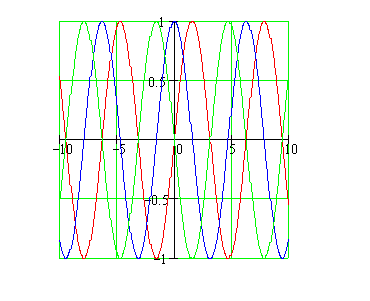Calculus on Demand at Dartmouth College Lecture 10 | Index | Lecture 12 Lecture 11Resources

Math 3 Course Syllabus
Practice Exams

Contents

In this lecture we continue our search for differentiation formulas by computing the derivatives of the standard trig functions.

Quick Question

What are the three functions whose graphs are shown?Outline

Outline for Derivatives of Trigonometric Functions

Textbook

Derivatives of Trigonometric Functions